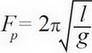# AP Physics 1 Question 15: Answer and Explanation

### Test Information

Question: 15

5. A ball of mass is attached to a string connected to the ceiling, forming a pendulum. When the string is raised to an angle of 50 degrees below the horizontal (the ceiling) and released, the ball swings back and forth with a period of 0.4 s. Which of the following changes to the pendulum would decrease the period of the pendulum?

• A. Increasing the mass of the ball
• B. Decreasing the length of the string
• C. Decreasing the angle of the string from the horizontal when released
• D. Decreasing the mass of the ball